Search

Exponential Functions: Compound Interest (page 4 of 5)

Sections: Introduction, Evaluation, Graphing, Compound interest, The natural exponentialOne very important exponential equation is the compound-interest formula: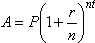...where "A" is the ending amount, "P" is the beginning amount (or "principal"), "r" is the interest rate (expressed as a decimal), "n" is the number of compoundings a year, and "t" is the total number of years.

Regarding the variables, n refers to the number of compoundings in any one year, not to the total number of compoundings over the life of the investment. If interest is compounded yearly, then n = 1; if semi-annually, then n = 2; quarterly, then n = 4; monthly, then n = 12; weekly, then n = 52; daily, then n = 365; and so forth, regardless of the number of years involved. Also, "t" must be expressed in years, because interest rates are expressed that way. If an exercise states that the principal was invested for six months, you would need to convert this to  6/12 = 0.5 years; if it was invested for 15 months, then t = 15/12 = 1.25 years; if it was invested for 90 days, then t = 90/365 of a year; and so on.

Note that, for any given interest rate, the above formula simplifies to the simple exponential form that we're accustomed to. For instance, let the interest rate r be 3%, compounded monthly, and let the initial investment amount be \$1250. Then the compound-interest equation, for an investment period of t years, becomes: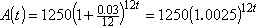...where the base is 1.0025 and the exponent is the linear expression 12t.

To do compound-interest word problems, generally the only hard part is figuring out which values go where in the compound-interest formula. Once you have all the values plugged in properly, you can solve for whichever variable is left.

• Suppose that you plan to need \$10,000 in thirty-six months' time when your child starts attending university. You want to invest in an instrument yielding 3.5% interest, compounded monthly. How much should you invest?
• To solve this, I have to figure out which values go with which variables. In this case, I want to end up with \$10,000, so A = 10,000. The interest rate is 3.5%, so, expressed as a decimal, r = 0.035. The time-frame is thirty-six months, so t = 36/12 = 3. And the interest is compounded monthly, so n = 12. The only remaining variable is P, which stands for how much I started with. Since I am trying to figure out how much to invest in the first place, then solving for P makes sense. I will plug in all the known values, and then I'll solve for the remaining variable: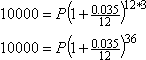The temptation at this point is to simplify on the right-hand side, and then divide off to solve for P. Don't do that; it tends toward round-off error, and can get you in trouble later on. Instead, stay exact, and do the dividing off symbolically (and exactly) first: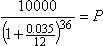Now I'll do the whole simplification in my calculator, working from the inside out, so everything is carried in memory and I get as exact an answer as possible: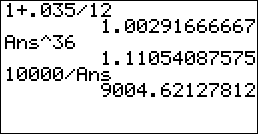I need to invest about \$9004.62.

(The problem did not specify how to round, but in this case, it didn't need to. Dollars-and-cents problems always round to two decimal places.)   Copyright © Elizabeth Stapel 2002-2011 All Rights Reserved

You should memorize the compound-interest formula, but you should also memorize the meaning of each of the variables in the formula. While you might be given the formula on the test, it is unlikely that you will be given the meanings of the variables, and, without the meanings, you will not be able to complete the exercises.

<< Previous  Top  |  1 | 2 | 3 | 4 | 5  |  Return to Index  Next >>

 Cite this article as: Stapel, Elizabeth. "Exponential Functions: Compound Interest." Purplemath. Available from     https://www.purplemath.com/modules/expofcns4.htm. Accessed [Date] [Month] 2016

Study Skills Survey

Tutoring from Purplemath
Find a local math tutor